Select Page

# Maths 12 Science CBSE Continuity and Differentiability Solutions for MCQ in English

Maths 12 Science CBSE Continuity and Differentiability Solutions for MCQ in English to enable students to get Solutions in a narrative video format for the specific question.

Expert Teacher provides Maths 12 Science CBSE Continuity and Differentiability Solutions for MCQ through Video Solutions in English language. This video solution will be useful for students to understand how to write an answer in exam in order to score more marks. This teacher uses a narrative style for a question from Continuity and Differentiability not only to explain the proper method of answering question, but deriving right answer too.

Please find the question below and view the Solution in a narrative video format.

Question:

Solution Video in English:

You can select video Solutions from other languages also. Please check Solutions in ( Hindi )

## Similar Questions from CBSE, 12th Science, Maths, Continuity and Differentiability

Question 1 :  Find the second order derivative of the function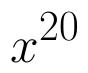. (View Answer Video)

Question 2 :  Find the second order derivative of the function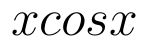. (View Answer Video)

Question 3 : Differentiate the function w.r.t.x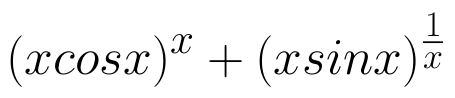. (View Answer Video)

Question 4 : Differentiate the function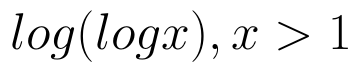with respect to x. (View Answer Video)

Question 5 : Differentiate the function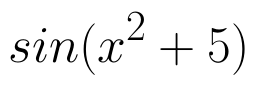with respect to x. (View Answer Video)

### Integrals

Question 1 :

Evaluate the following definite integral :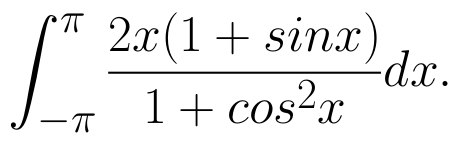(View Answer Video)

Question 2 : Evaluate: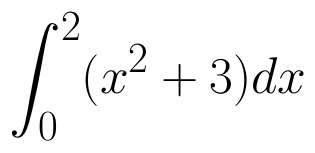as limit of sums.  (View Answer Video)

Question 3 : Find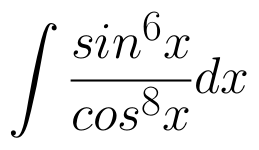. (View Answer Video)

Question 4 : Show that :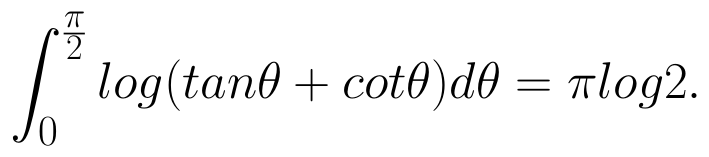(View Answer Video)

Question 5 : Evaluate :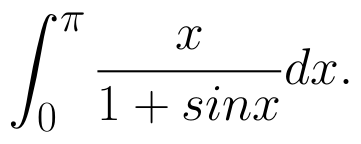(View Answer Video)

### Application of Integrals

Question 1 : Find the area of the region bounded by the parabola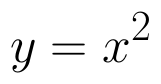and y = | x |. (View Answer Video)

Question 2 : Find the area enclosed by the parabola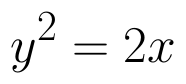and the line x - y = 4. (View Answer Video)

Question 3 : Using integration, find the area of the region bounded by the curvesand y = x. (View Answer Video)

Question 4 : Using the method of integration find the area of the region bounded by lines:
2x + y = 4, 3x - 2y = 6 and x - 3y + 5 = 0.
Question 5 : Find the area enclosed between the parabola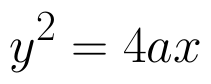and the line y = mx. (View Answer Video)